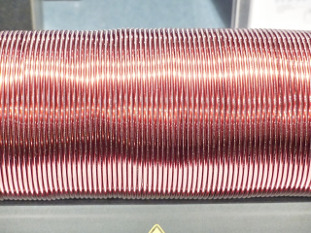# Conduction

In other languages: FrançaisFigure 1. Common metals, such as copper, display both thermal and electrical conductivity.

Conduction can refer to either:

The word conductor usually means a material with high electrical conductivity (low resistivity). However, most electric conductors (usually metals), are good thermal conductors as well. For example, copper is both an excellent thermal conductor and electrical conductor. A simple model that explains this relationship (a good conceptual model, but it skips some important details) is pretending that the electrons in the metal behave like a gas that are free to move and carry both electric current and heat.

## Ratio of Thermal to Electrical Conductivity

The values for thermal conductivity and electrical conductivity for many metals depend on temperature. The higher the temperature the greater the thermal conduction (compared to electrical conduction). This linear relationship can be expressed and compared using a ratio known as the Wiedemann-Franz law. This ratio is expressed as:

Where:

• is a constant known as the Lorentz number, equal to
• is the thermal conductivity of the material
• is the electrical conductivity of the material
• is the temperature of the material, in Kelvin
• is the Boltzmann's constant
• is the elementary charge of an electron

The reverse is usually but not always true; for example, diamonds are excellent thermal conductors (even better than copper) but usually electrical insulators. Although at very low temperatures a research group found  showing that diamonds can become superconductors below 4 K (superconductivity is specifically a description of electrical conduction, not thermal conduction).